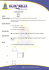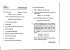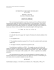# 10th Grade | Unit 5

## Transcription

10th Grade | Unit 5
```MATH
STUDENT BOOK
10th Grade | Unit 5
Unit 5 | Similar Polygons
MATH 1005
Similar Polygons
INTRODUCTION |3
1. PRINCIPLES OF ALGEBRA
5
RATIOS AND PROPORTIONS |5
PROPERTIES OF PROPORTIONS |11
SELF TEST 1 |16
2.SIMILARITY
18
MEANING OF SIMILARITY |18
THEOREMS ABOUT SIMILAR POLYGONS |31
THEOREMS ABOUT SIMILAR TRIANGLES |36
SELF TEST 2 |61
3. RIGHT TRIANGLES
64
GEOMETRY |64
TRIGONOMETRY |69
INDIRECT MEASURE |79
SELF TEST 3 |85
GLOSSARY |89
LIFEPAC Test is located in the
center of the booklet. Please
remove before starting the unit.
Section 1 |1
Similar Polygons | Unit 5
Author:
Milton R. Christen, M.A.
Editor-in-Chief:
Richard W. Wheeler, M.A.Ed.
Editor:
Robin Hintze Kreutzberg, M.B.A.
Consulting Editor:
Robert L. Zenor, M.A., M.S.
Revision Editor:
Alan Christopherson, M.S.
804 N. 2nd Ave. E.
Rock Rapids, IA 51246-1759
© MCMXCVII by Alpha Omega Publications a division of
trademark of Alpha Omega Publications, Inc.
All trademarks and/or service marks referenced in this material are the property of their respective
owners. Alpha Omega Publications, Inc. makes no claim of ownership to any trademarks and/
or service marks other than their own and their affiliates, and makes no claim of affiliation to any
companies whose trademarks may be listed in this material, other than their own.
2| Section 1
Unit 5 | Similar Polygons
Similar Polygons
Introduction
In our study of congruent triangles, we learned that congruent triangles have the same size and the same
shape. We are now going to study objects that have the same shape, but not necessarily the same size.
We have all looked at photographs. The photograph shows a smaller or larger version of the object that was
photographed. The picture is the same shape but a different size.
We have all taken trips and used a road map to help get us from here to there. A map is a smaller version of
the real thing.
Some of you may have built model airplanes or may have a model train. These models are scaled-down
versions of a real item. The model is the same shape as the real thing but the size is different.
These examples are all practical examples of similarly shaped objects. In mathematics we also study similar
shapes. In this LIFEPAC® we shall learn and use some principles of algebra in our study of similarity and its
application to the right triangle.
Objectives
Read these objectives. The objectives tell you what you will be able to do when you have successfully
completed this LIFEPAC. When you have finished this LIFEPAC, you should be able to:
1. Write ratios in simplest form.
2. Name the properties of proportions.
3. Solve proportion problems.
4. Identify similar polygons by using definitions, postulates, and theorems.
5. Use theorems about special segments in similar triangles.
6. Solve problems about similar right triangles.
7. Use the Pythagorean Theorem.
8. Solve triangle problems using trigonometry.
9. Find measurements indirectly.
Section 1 |3
Similar Polygons | Unit 5
________________________________________________________________________________________________ ________________________________________________________________________________________________
________________________________________________________________________________________________
________________________________________________________________________________________________
________________________________________________________________________________________________
________________________________________________________________________________________________
________________________________________________________________________________________________
________________________________________________________________________________________________
________________________________________________________________________________________________
________________________________________________________________________________________________
________________________________________________________________________________________________
________________________________________________________________________________________________
________________________________________________________________________________________________
________________________________________________________________________________________________
________________________________________________________________________________________________
________________________________________________________________________________________________
________________________________________________________________________________________________
________________________________________________________________________________________________
________________________________________________________________________________________________
4| Section 1
Unit 5 | Similar Polygons
1. PRINCIPLES OF ALGEBRA
Before we learn and use some of the properties of similar polygons, we should review some basic
principles from algebra.
Section Objectives
Review these objectives. When you have completed this section, you should be able to:
1. Express ratios in simplest form.
2. Name the properties of proportion.
3. Solve proportion problems.
RATIOS AND PROPORTIONS
Two ideas from algebra that we need to review
at this time are ratios and proportions.
DEFINITION
Ratio: the comparison of two numbers by
division. The quotient is the ratio of the
two numbers.
1
4
The ratio of 3 to 15 is 5. The ratio of 8 to 2 is 1.
a
The ratio of a to b is b. Notice the quotients,
1 4 a
5, 1, b, are written as fractions. We can arrive at
the ratio by dividing the “to” number into the
“of” number. Keep in mind that you are not
finding the ratio of one object to another, but
rather the ratio of two numbers that are measures of the object in the same unit.
Rather than go through a division process to
find a ratio, we can set up the two numbers as
a fraction and reduce the fraction.
The “of” number will be the numerator (top)
and the “to” number will be the denominator
(bottom).
Model 1: Find the ratio of 6 to 8.
6
8
3
=4
Model 2: If AB = 6 inches and CD =
18 inches, find the ratio of
AB to CD.
AB 6
CD = 18
1
=3
Model 3: If +A = 35°, +B = 50°, find the
ratio of +A to +B.
A 35
B = 50
Model 4:
If the side of one triangle is
2 feet and the side of another
triangle is 18 inches, find the
ratio of the small side to the
large side.
18
7
= 10
3
24 = 4 1
1 2
3
2
(2 feet was changed to
24 inches)
3
2 = 2 = 4 (18 inches was changed
1
to 12 feet)
Ratio is simply a number. No units are connected to a ratio.
Section 1 |5
Similar Polygons | Unit 5
The ratio of two numbers can also be written in
the form a:b. This form is useful when we are
comparing three or more numbers.
The following sets of numbers all have the ratio
3:4:5.
{12, 16, 20}
Model 5:
The ratio of 3 to 4 to 5 can be
written as 3:4:5.
This ratio means that the ratio of the first to the second is 43, the ratio {15x2, 20x2, 25x2}
of the second to the third is 54, and
the ratio of the first to the third is 35.
12
16
3 16
4 12
3
3 4x
4 3x
3
= 4, 20 = 5, 20 = 5
3x
{3x, 4x, 5x} 4x = 4, 5x = 5, 5x = 5
2
15x
20x2
3 20x2 4 15x2 3
= 4, 25x2 = 5, 25x2 = 5
Express each ratio in simplest form.
1.1
6
12
=
__________________
1.2 9
6
1.3
18
29
=
__________________
1.4
1.5 21:28
=
__________________
1.6 25:45
1.7 10:20
=
__________________
=
__________________
=
__________________
=
__________________
1.8 15:25:35 =
__________________
36
72
If a = 3 and b = 5, find each ratio.
__________________
1.10
b
a =__________________
1.11(a + b)= __________________
1.12
b
(a + b)=
__________________
1.14
d to c=
__________________
1.16
(d – c)
(d + c)=
__________________
1.9
a
b
=
a
If c = 4 and d = 5, find each ratio.
1.13c to d= __________________
d
1.15(c + d)= __________________
6| Section 1
Unit 5 | Similar Polygons
Use the following figures to find each ratio in simplest form.
A
12
P
14
15
6
D
E
O
6
7
9
B
C
Q
1.17
DB=
__________________
1.18
AB=
__________________
1.19
DB
AB=
__________________
1.20
AE
AC=
__________________
1.21
AC
AE= __________________
1.22
EC
AC=
__________________
S
R
P
14
10
15
6
E
O
7
9
C
10
Q
S
1.23
PO
OS=
__________________
1.24
PO
PS= __________________
1.25
OS
PS= __________________
1.26
OR
QO=
1.27
OR
QR=
1.28
QR
PS= __________________
__________________
__________________
Work the following problems.
1.29
The measures of the angles of a triangle are in the ratio of 1:2:3. Find the measure
of each angle. (Hint: The sum of the angles of a triangle = 180°.)
1.30
The distance from A to B is 60 feet. The distance from B to C is 10 yards. The
distance from C to D is 20 inches. Find the ratio of AB:BC:CD.
Section 1 |7
Similar Polygons | Unit 5
DEFINITION
Proportion: an equation that states
that two ratios are equal.
a c The proportion b = d tells us that the ratio a to b
and the ratio c to d are equal ratios. The proportion can be read, a is to b as c is to d; or, the
quotient of a and b equals the quotient of c and
d. The proportion can also be written a:b = c:d.
Each of the four numbers a, b, c, and d is called
a term of the proportion: a is the first term, b
is the second term, c is the third term, and d is
the fourth term.
The first and the fourth terms are known as the
extremes of the proportion. The second and
third terms are called the means.
3:4
means
3
The fact that more than two ratios are equal is
often expressed in the form of an extended
proportion.
a c e b=d=f
1
2
2
=
4
___
3
4 5
6
7
8
= 4 = 6 = 8 = 10 = 12 = 14 = 16.
We can pick any two of the ratios and form a
6 7
regular proportion: 63 = 12
, 14 = 42.
Since a proportion is an equation, we can use
the properties of equality to transform a pro a c portion to another form. For example, b = d can
be written as ad = bc by using the multiplication
property of equality (multiply each side of the
equation by bd).
= 15:20
15
___
g = h is an extended proportion stating that all four ratios are the same number.
Another extended proportion is
means
a
= c
b
d
(bd ) a = (bd ) c
b
d
20
extremes
extremes
Name the means and the extremes in these proportions.
1.31
1.32
3
4
5
7
15
= 20
20
= 28
6 x means: ____________________
extremes: ____________________
means: ____________________
extremes: ____________________
1.33
11
= y
means: ____________________
extremes: ____________________
1.34
3:9 = 2:6
means: ____________________
extremes: ____________________
1.35
1:2 = 4:8
means: ____________________
extremes: ____________________
1.36
x:y = 3:7
means: ____________________
extremes: ____________________
8| Section 1
Unit 5 | Similar Polygons
Find the value of x in each of these proportions.
1.37
x
25
= 5
2
x=
______________
1.38
x
6
= 2
x=
______________
1.39
9
3
x = 12
x=
______________
1.40
10
x
7 = 5
x=
______________
1.41
9:x = x:4
x=
______________
1.42
1
2 : x
x=
______________
1.43
(x + 3)
6
x=
______________
1.44
(x + 1)
(x + 2)
x=
______________
1.45
3
2
x=
______________
5
= 4
x
= 4
3
= 23 : 43
2
= 3
Find the ratio of x to y.
1.462x = 3y
y
_________________
1.475x = 7y
_________________
_________________
1.492x – 3y = 0
_________________
1.48
x
3
1.50
x – 5y = 0 _________________
= 2
Along with our knowledge of complementary and supplementary angles, ratios can help us solve
geometry problems.
Model:
Two complementary angles have measures in the ratio of 4 to 5.
Find the measure of each angle.
Let 4x = measure of the smaller angle.
Solution:
5x = measure of the larger angle.
Then 4x + 5x =
90°
9x
=
90°
x
=
10°
4x
=
40°
5x
=
50°
(angles are complementary)
Section 1 |9
Similar Polygons | Unit 5
Solve the following problems. Show your work and circle your answer.
1.51 Two complementary angles have measures in the ratio of 1 to 5. Find the measure of each
angle.
1.52 The ratio of the measures of two supplementary angles is 3:7. Find the measure of each
angle.
1.53
A 30-inch segment is cut into two parts whose lengths have the ratio 3 to 5. Find the length
of each part.
1.54
The perimeter of a triangle is 48 inches and the sides are in the ratio of 3:4:5. Find the
length of each side.
1.55
A triangle has a perimeter of 18 inches. If one side has a length of 8 inches, find the other
two sides if their lengths are in the ratio of 23.
10| Section 1
Unit 5 | Similar Polygons
PROPERTIES OF PROPORTIONS
You will often wish to change a proportion into
some equivalent equation. Although we can
make this change by using basic properties of
algebra, we will save time and steps by using
some special properties of proportions. When
we use these properties for a reason on a
proof, we shall simply write POP (Property of
Proportion).
In these statements the variables used represent nonzero numbers.
EQUIVALENT FORMS PROPERTY
a
b
c a
b d
c b
d
= d , c = d , b = a , a = c are equivalent
proportions. The cross product of each
proportion gives the same equation,
Model 1:
2
5
4=10
20=20
10
5
4=2
CROSS PRODUCT PROPERTY
If
a c b = d,
then ad = bc.
Model 1:
3
15
5=25
Model 2:
3 • 25 = 5 • 15
75=75
Model 3:
Model 2:
Model 3:
20=20
2
4
5=10
20=20
6
12
11=22
6 • 22 = 11 • 12
132=132
Model 4:
4
10
2=5
20=20
x
3
10= 5
5x=30
x=6
Section 1 |11
Similar Polygons | Unit 5
DENOMINATOR SUM PROPERTY
If
a c b = d,
then
(a + b)
b
=
DENOMINATOR DIFFERENCE PROPERTY
(c + d)
d .
If
We can add 1 to both sides of the equation:
a c b=d
a b
a b c d b + b=d + d
Model 1:
+ 1
(a + b)
=
c d
+1
(c + d)
b=d
2
5
4=10
(2 + 4)
(5 + 10)
4=10
6
15
4=10
3
1
9=3
Model 2:
(3 + 9)
(1 + 3)
9=3
12
4
9=3
2
3
8=12
Model 3:
(2 + 8)
(3 + 12)
8=12
10
15
8=12
12| Section 1
a c b = d,
then
(a – b)
b
=
(c – d)
d
.
We can subtract 1 from both sides of the
equation:
a c b=d
a b –
a b c d b – b=d – d
(a – b)
(c – d)
b=d
Model 1:
1
=
c d –
1
8
16
3=6
(8 – 3)
(16 – 6)
3=6
5
10
3=6
12
4
3=1
Model 2:
(12 – 3)
(4 – 1)
3=1
9
3
3=1
6
3
8=4
Model 3:
(6 – 8)
(3 – 4)
8=4
– 8=– 4
2
1
Unit 5 | Similar Polygons
NUMERATOR-DENOMINATOR SUM PROPERTY
If
Let
a c e g b = d = f = h =
a b=
c ..., then
(a + c + e + g + ...)
(b + d + f + h + ...)
=
a
b
c =d=
...
e x, d = x, f = x, ...
(a + c + e + ...)
(b + d + f + ...)
=
4
8
(2 + 4 + 6 + 8) =
1
2
then a = bx, c = dx, e = fx, ...
so
e f
Model 1:
1
2
=
2
4
=
3
6
(1 + 2 + 3 + 4)
(bx + dx + fx + ...)
10
=(b + d + f + ...)
20 =
1
2
(b + d + f + ...) =
x (b + d + f + ...)
=
x
=
a
b
=
Model 2:
3
5
=
6
10
=
(3 + 6 + 9)
c d
=
e f
9
15
(5 + 10 + 15) =
...
18
30 =
6
10
6
10
Name the POP illustrated.
1.56If
a 2
b 1.57
If
(a + 1)
3
1.58
If
x y
=
1.59
If
x 4
= 3 , then
1.60
If
1.61
If
= 5 , then
r s
r x
=
(b + 5)
5 .
______________________________________
b = 5 , then 5(a + 1) = 3b.
t = u , then
y (x + 3)
2
(a + 2)
2
=
x y
(y + 6)
3 =
(x + r + t)
(y + s + u)
=
x y .
______________________________________
______________________________________
4
3 .______________________________________
, then
(x + 1)
2
=
(y + 3)
3
.
______________________________________
s = t , then sx = rt.______________________________________
Section 1 |13
Similar Polygons | Unit 5
Given xy = ab, form a proportion in which:
1.62
x is the first term.
____________________________________________________
1.63
x is the second term.
____________________________________________________
1.64
a is the second term.
____________________________________________________
1.65
b is the first term.
____________________________________________________
1.66
y is one of the extremes.
____________________________________________________
Find x and y in each proportion.
1.67
2 x
=
5
y
= 5 x = _________________
3
1.68
2 x
=
y 4
= 4x = _________________
1.69
2 3
=
x 6
= yx = _________________
1
9 y = _________________
y = _________________
y = _________________
Find the fourth term of a proportion when the first three terms are:
1.70
2, 3, and 4.
______________________________
1.71
6, 2, and 8.
______________________________
1.72
1 2
2, 3,
3
and 4 .______________________________
Find the value of a in each proportion.
1.73
(a + 1)
4
= 3 a = _________________
1.74
(a – 2)
3
= 4 a = _________________
1.75
a 9 4 = a a
14| Section 1
2 a = _________________
Unit 5 | Similar Polygons
BX
Given XC
AB
= AC
, complete the following proportions.
1.76
BX BA=
__________________________
1.77
BX + XC XC=
__________________________
1.78
AC XC=
__________________________
1.79
AC AB=
__________________________
AX
Given XB
AY
= YC
A
B
X
C
, find the missing lengths.
AXXBAYYC
A
1.80
346
___________
1.81
5
4___________6
1.82
___________
448
1.83
If
a
b
=
5
7
and
7 ___________14
c d
=
3
4,
1.84
d c
1.86
(a + b)
a=
1.88
2c 3d=
Y
X
B
C
4
find each ratio in simplest form.
1.85
(a + b)
b=
_______________________
_______________________
1.87
(c + d)
c=
_______________________
_______________________
1.89
d c
=_______________________
=_______________________
TEACHER CHECK
initials
date
Review the material in this section in preparation for the Self Test. The Self Test will
check your mastery of this particular section. The items missed on this Self Test will indicate specific areas where restudy is needed for mastery.
Section 1 |15
Similar Polygons | Unit 5
SELF TEST 1
Complete the following statements (each answer, 3 points).
1.01
A ratio compares two numbers by __________________________ .
1.02
The ratio of 3 to 4 is written as __________________________ .
1.03
Another way of writing the ratio of 3 to 4 is __________________________ .
1.04
In writing the ratio of measurement numbers, the units must be the ______________________ .
1.05
The ratio of 3 to 4 and the ratio of 4 to 3 (are/are not) ________________ the same number.
1.06
A proportion is an equation that states two __________________________ are equal.
1.07
“If
2
3
1.08
“If
x 2
y=3
1.09
In the proportion b = q , the third term is __________________________ .
x = y , then 2y = 3x ” is an example of the __________________________ POP.
a (x + 2 + a)
2
= b , then (y + 3 + b) = 3 ” is an example of the __________________________ POP.
a p 5
10
1.010 In the proportion 6 = 12 , the means are a. ________________ and b. ________________ .
Express each ratio in simplest form (each answer, 2 points).
1.011 Ratio of 6 feet to 3 feet.
________________________________
1.012 Ratio of 7 yards to 6 feet.
________________________________
1.013 Ratio of 12 to 100.
________________________________
A
B
}
}
3
C
9
1.014 Ratio of AB to BC.________________________________
1.015 Ratio of BC to AC.________________________________
16| Section 1
Unit 5 | Similar Polygons
Find x in the following proportions (each answer, 2 points).
1.016
x 7
1.018
5
2x
3
x = ______________
1.017 3:8 = x:32
25
4
x = ______________
1.019
x 3
x x = ______________
1.021
x+2
2 = 5
=
16
1.020 x = 4
=
x+2
5
=
6+2
2
x = ______________
x = ______________
x = ______________
Find the required numbers (each answer, 2 points).
TU UW
WT
Given:
TR = RS = ST
T
TU = 3
RS = 15
TW = 4
TR = 9
1.022 UW= ______________
U
W
R
S
1.023 ST =______________
1.024 WS=______________
1.025 UR=______________
54
67
SCORE
TEACHER
initials
date
Section 1 |17
MAT1005 – Apr ‘15 Printing
804 N. 2nd Ave. E.
Rock Rapids, IA 51246-1759
800-622-3070
www.aop.com
ISBN 978-0-86717-635-3
9 780867 176353
```

### HSM12CC_GM_07_02_CM - Newark Catholic High School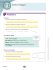### 7-2 Practice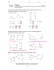### STANDARD NORMAL DISTRIBUTION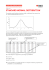### STANDARD NORMAL DISTRIBUTION### Electrical Basic of Electrical Engineering, Power System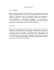### 12 Solving Percents Using Proportions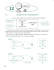### 2010 SOL Geometry Released Test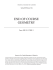### to View the File - Blue Bells Public School Junior Section